# نتائج البحث (16)

View
Selected filters:
• Statistics and ProbabilityConditions of Use:
Remix and Share
Rating

This learning video deals with a question of geometrical probability. A key idea presented is the fact that a linear equation in three dimensions produces a plane. The video focuses on random triangles that are defined by their three respective angles. These angles are chosen randomly subject to a constraint that they must sum to 180 degrees. An example of the types of in-class activities for between segments of the video is: Ask six students for numbers and make those numbers the coordinates x,y of three points. Then have the class try to figure out how to decide if the triangle with those corners is acute or obtuse.

Subject:
Geometry
Statistics and Probability
Material Type:
Lecture
Provider:
MIT
Provider Set:
MIT Blossoms
Author:
Gilbert Strange
06/02/2012Conditions of Use:
Remix and Share
Rating

This task was developed by high school and postsecondary mathematics and design/pre-construction educators, and validated by content experts in the Common Core State Standards in mathematics and the National Career Clusters Knowledge & Skills Statements. It was developed with the purpose of demonstrating how the Common Core and CTE Knowledge & Skills Statements can be integrated into classroom learning - and to provide classroom teachers with a truly authentic task for either mathematics or CTE courses.

Subject:
Architecture and Design
Geometry
Statistics and Probability
Material Type:
Activity/Lab
Assessment
Homework/Assignment
Lesson Plan
Provider:
National Association of State Directors of Career Technical Education Consortium
Provider Set:
Career Technical Education
03/05/2012Conditions of Use:
Remix and Share
Rating

This task was developed by high school and postsecondary mathematics and health sciences educators, and validated by content experts in the Common Core State Standards in mathematics and the National Career Clusters Knowledge & Skills Statements. It was developed with the purpose of demonstrating how the Common Core and CTE Knowledge & Skills Statements can be integrated into classroom learning - and to provide classroom teachers with a truly authentic task for either mathematics or CTE courses.

Subject:
Health, Medicine and Nursing
Algebra
Ratios and Proportions
Statistics and Probability
Material Type:
Activity/Lab
Lesson Plan
Provider:
National Association of State Directors of Career Technical Education Consortium
Provider Set:
Career Technical Education
07/27/2012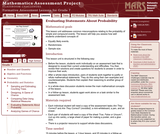Rating

This lesson unit addresses common misconceptions relating to probability of simple and compound events. The lesson will help you assess how well students understand concepts of: Equally likely events; randomness; and sample sizes.

الموضوع:
الإحصائيات والاحتمالات
نوع المادة:
التقييم
Lesson Plan
Provider:
Shell Center for Mathematical Education
U.C. Berkeley
Provider Set:
Mathematics Assessment Project (MAP)
Mathematics Assessment Project (MAP)
04/26/2013Conditions of Use:
Read the Fine Print
Rating

This web site offers families, teachers, and tutors 80 mathematical challenges helpful for encouraging problem solving with students in grades 6 to 8. The math challenges focus on concepts and objects found in everyday life, such as how fast your heart beats, what shape container holds the most popcorn, and how much of me shows in a mirror. Each challenge contains an initial problem with a solution hint, a complete explanation of the answer, and additional problems related to the same challenge. Resources for further investigations are suggested as well. From the Printing the Challenges link on the homepage, PDF files are available for all 80 challenges in English, the first 15 challenges in Spanish, and the family resource materials in English and Spanish.

Subject:
Algebra
Geometry
Statistics and Probability
Material Type:
Activity/Lab
Homework/Assignment
Provider:
National Council of Teachers of Mathematics
Provider Set:
Figure This!
01/01/2002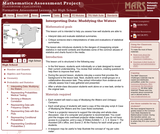Rating

This lesson unit is intended to help teachers assess how well students are able to: interpret data and evaluate statistical summaries; and critique someone elseŐs interpretations of data and evaluations of statistical summaries. The lesson also introduces students to the dangers of misapplying simple statistics in real-world contexts, and illustrates some of the common abuses of statistics and charts found in the media.

الموضوع:
الإحصائيات والاحتمالات
نوع المادة:
التقييم
Lesson Plan
Provider:
Shell Center for Mathematical Education
U.C. Berkeley
Provider Set:
Mathematics Assessment Project (MAP)
Mathematics Assessment Project (MAP)
04/26/2013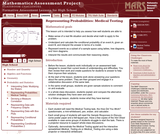Rating

This lesson unit is intended to help teachers assess how well students are able to: make sense of a real life situation and decide what math to apply to the problem; understand and calculate the conditional probability of an event A, given an event B, and interpret the answer in terms of a model; represent events as a subset of a sample space using tables, tree diagrams, and Venn diagrams; and interpret the results and communicate their reasoning clearly.

الموضوع:
الإحصائيات والاحتمالات
نوع المادة:
التقييم
Lesson Plan
Provider:
Shell Center for Mathematical Education
U.C. Berkeley
Provider Set:
Mathematics Assessment Project (MAP)
Mathematics Assessment Project (MAP)
04/26/2013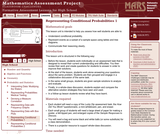Rating

This lesson unit is intended to help teachers assess how well students are able to: Understand conditional probability; represent events as a subset of a sample space using tables and tree diagrams; and communicate their reasoning clearly.

الموضوع:
الإحصائيات والاحتمالات
نوع المادة:
التقييم
Lesson Plan
Provider:
Shell Center for Mathematical Education
U.C. Berkeley
Provider Set:
Mathematics Assessment Project (MAP)
Mathematics Assessment Project (MAP)
04/26/2013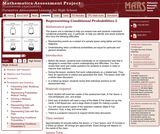Rating

This lesson unit is intended to help teachers assess how well students understand conditional probability, and, in particular, to help teachers identify and assist students who have the following difficulties: representing events as a subset of a sample space using tables and tree diagrams; and understanding when conditional probabilities are equal for particular and general situations.

الموضوع:
الإحصائيات والاحتمالات
نوع المادة:
التقييم
Lesson Plan
Provider:
Shell Center for Mathematical Education
U.C. Berkeley
Provider Set:
Mathematics Assessment Project (MAP)
Mathematics Assessment Project (MAP)
04/26/2013Conditions of Use:
بلا تقييدات
Rating

In this group task students collect data and analyze from the class to answer the question "is there an association between whether a student plays a sport and whether he or she plays a musical instrument? "

الموضوع:
الرياضيات
الإحصائيات والاحتمالات
نوع المادة:
Activity/Lab
Provider:
Illustrative Mathematics
Provider Set:
Illustrative Mathematics
المؤلف:
Illustrative Mathematics
10/09/2012Conditions of Use:
Read the Fine Print
Rating

These data analysis problems of the month are designed to be used schoolwide to promote a problem-solving theme at your school. Each problem is divided into five levels, Level A through Level E, to allow access and scaffolding for the students into different aspects of the problem and to stretch students to go deeper into mathematical complexity. The problems cover statistics, probability, discrete math, and counting principles.

Subject:
Statistics and Probability
Material Type:
Activity/Lab
Provider:
Noyce Foundation
Provider Set:
Inside Mathematics
11/30/2011Conditions of Use:
قراءة النص المطبوع بخط رفيع
Rating

These data analysis problems of the month are designed to be used schoolwide to promote a problem-solving theme at your school. Each problem is divided into five levels, Level A through Level E, to allow access and scaffolding for the students into different aspects of the problem and to stretch students to go deeper into mathematical complexity. The problems cover statistics, probability, discrete math, and counting principles.

الموضوع:
الإحصائيات والاحتمالات
نوع المادة:
Activity/Lab
Provider:
Noyce Foundation
Provider Set:
في قلب الرياضيات
11/30/2011Conditions of Use:
قراءة النص المطبوع بخط رفيع
Rating

These data analysis problems of the month are designed to be used schoolwide to promote a problem-solving theme at your school. Each problem is divided into five levels, Level A through Level E, to allow access and scaffolding for the students into different aspects of the problem and to stretch students to go deeper into mathematical complexity. The problems cover statistics, probability, discrete math, and counting principles. Level A and B are lower and upper primary school levels, while C and D are for middle school and early high school students. Level E problems are for upper level high schoolers.

الموضوع:
الإحصائيات والاحتمالات
نوع المادة:
Activity/Lab
Provider:
Noyce Foundation
Provider Set:
في قلب الرياضيات
11/30/2011Conditions of Use:
قراءة النص المطبوع بخط رفيع
Rating

These data analysis problems of the month are designed to be used schoolwide to promote a problem-solving theme at your school. Each problem is divided into five levels, Level A through Level E, to allow access and scaffolding for the students into different aspects of the problem and to stretch students to go deeper into mathematical complexity. The problems cover statistics, probability, discrete math, and counting principles.

الموضوع:
الإحصائيات والاحتمالات
نوع المادة:
Activity/Lab
Provider:
Noyce Foundation
Provider Set:
في قلب الرياضيات
11/30/2011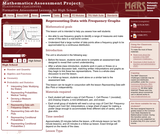Rating

This lesson unit is intended to help teachers assess how well students: are able to use frequency graphs to identify a range of measures and make sense of this data in a real-world context; and understand that a large number of data points allow a frequency graph to be approximated by a continuous distribution.

Subject:
Measurement and Data
Statistics and Probability
Material Type:
Assessment
Lesson Plan
Provider:
Shell Center for Mathematical Education
U.C. Berkeley
Provider Set:
Mathematics Assessment Project (MAP)
Mathematics Assessment Project (MAP)
04/26/2013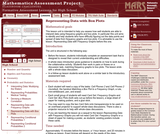Rating

This lesson unit is intended to help teachers assess how well students are able to interpret data using frequency graphs and box plots. In particular this unit aims to identify and help students who have difficulty figuring out the data points and spread of data from frequency graphs and box plots. It is advisable to use the lesson: Representing Data 1: Frequency Graphs, before this one.

Subject:
Measurement and Data
Statistics and Probability
Material Type:
Assessment
Lesson Plan
Provider:
Shell Center for Mathematical Education
U.C. Berkeley
Provider Set:
Mathematics Assessment Project (MAP)
Mathematics Assessment Project (MAP)